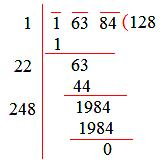# How to solve square root in division method?

Step I: Group the digits in pairs, starting with the digit in the units place. Each pair and the remaining digit (if any) is called a period.

Step II: Think of the largest number whose square is equal to or just less than the first period. Take this number as the divisor and also as the quotient.

Step III: Subtract the product of the divisor and the quotient from the first period and bring down the next period to the right of the remainder. This becomes the new dividend.

Step IV: Now, the new divisor is obtained by taking two times the quotient and annexing with it a suitable digit which is also taken as the next digit of the quotient, chosen in such a way that the product of the new divisor and this digit is equal to or just less than the new dividend.

Step V: Repeat steps (2), (3), and (4) until all the periods have been taken up. Now, the quotient so obtained is the required square root of the given number.

Example: